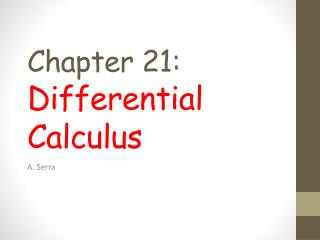DownloadDownload PresentationChapter 21: Differential Calculus

# Chapter 21: Differential Calculus

Download Presentation## Chapter 21: Differential Calculus

- - - - - - - - - - - - - - - - - - - - - - - - - - - E N D - - - - - - - - - - - - - - - - - - - - - - - - - - -
##### Presentation Transcript

1. Chapter 21:Differential Calculus A. Serra

2. A. Rates of change Class work: A rate as the comparison between two quantities of different kinds. Review 8C (Gradient) from previous year. Exercise 21A.1 4 page 627 Individual work: • Examples 1,2: Do them & check your answers. • Exercise 21A1: 1,3 • Exercise 21A2: 1 (quiz next lesson?) • Extra: 21A: even numbers • Extra. Investigation: constant and variable rates of change. Page 627.

3. B. Limits. CLASS WORK Correct exercise 21A.2 page 629 Rate of change vs. instantaneous rate of change. The instantaneous rate of change of a variable at a particular instant is given by the gradient of the tangent to the graph at that point. Example 21A.2 page 629 estimating and limits…(the foundation of differential calculus). Example limits Exercise 21B 1a, 2a INDIVIDUAL WORK • Example 3: Do it & check your answers. • Exercise 21A.3: 1 • Exercises 21B 1b,2b. If you cannot do them independently ask for help and do 21B 1c,2c • Extra: Other exercises in 21B

4. C. The derivative function. CLASS WORK With the slope function f’(x) (also known as the derived function or derivative function or the derivative), we will be able to find the slope of the tangent at any point (x, f(x)). f’(x) “f dashed x” = dy/dx “dee y by dee x” GeoGebra: Slope and derivative of a function Group work: Investigation: The derivative of y=x^2 (page 635) INDIVIDUAL WORK • Example 4: Do it & check your answers. • Exercise 21C: 1. • Extra: Other exercises in 21C • Extra: Investigation “Simple rules of differentiation” (page 638) (presentation?)

5. D. Simple rules of differentiation. Differentiation is the process of finding a derivative or gradient function. CLASS WORK Discoveries: The derivative of y=x^n (from results homework Exercise 21C1). Simple rules of differentiation (from investigation volunteers). INDIVIDUAL WORK • Examples 5, 6, 7: Do them & check your answers. • Exercise 21D: 1,3, 5 • Extra: Other exercises in 21D.

6. E. Tangents to curves. CLASS WORK Review 8F (Equations of lines). Now m= f’(a) = (y-b)/(x-a) Exercise 21E:1 Students pick 2 letters. INDIVIDUAL WORK • Examples 8& 9: Do them & check your answers. • Exercises 21E: 1 (two done in class) • Extra: 21E any other exercise.

7. F. The second derivative. The second derivative is the derivative of the first derivative  CLASS WORK F double dashed of x = dee two y by dee x squared” Example s= 2t^3+12t^2+24t >> v >>> a INDIVIDUAL WORK • Example 10: Do it & check your answers. • Exercises 21F: two of the following: 1 (easy but long), 3, 4 • Extra: 21F any other exercise.

8. Review • INDIVIDUAL WORK • Review Set 21A. • Extra: Review Set 21B and/or 21C NEXT • Units 22 and 23…. • Quizzes and tests • Good luck 